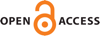Download this articleFor screen For printingRecent IssuesThe Journal About the Journal Editorial Board Editors’ Interests Subscriptions Submission Guidelines Submission Form Policies for Authors Ethics Statement ISSN: 1948-206X (e-only) ISSN: 2157-5045 (print) Author Index To Appear Other MSP JournalsResonances for Schrödinger operators on infinite cylinders and other products

### T. J. Christiansen

Vol. 16 (2023), No. 7, 1497–1546##### Abstract

We study the resonances of Schrödinger operators on the infinite product $X={ℝ}^{d}×{\mathbb{𝕊}}^{1}$, where $d$ is odd, ${\mathbb{𝕊}}^{1}$ is the unit circle, and the potential $V$ lies in ${L}_{c}^{\infty }\left(X\right)$. This paper shows that at high energy, resonances of the Schrödinger operator $-\mathrm{\Delta }+V$ on $X={ℝ}^{d}×{\mathbb{𝕊}}^{1}$ which are near the continuous spectrum are approximated by the resonances of $-\mathrm{\Delta }+{V}_{0}$ on $X$, where the potential ${V}_{0}$ is given by averaging $V$ over the unit circle. These resonances are, in turn, given in terms of the resonances of a Schrödinger operator on ${ℝ}^{d}$ which lie in a bounded set. If the potential is smooth, we obtain improved localization of the resonances, particularly in the case of simple, rank $1$ poles of the corresponding scattering resolvent on ${ℝ}^{d}$. In that case, we obtain the leading order correction for the location of the corresponding high-energy resonances. In addition to direct results about the location of resonances, we show that at high energies away from the resonances, the resolvent of the model operator $-\mathrm{\Delta }+{V}_{0}$ on $X$ approximates that of $-\mathrm{\Delta }+V$ on $X$. If $d=1$, in certain cases this implies the existence of an asymptotic expansion of solutions of the wave equation. Again for the special case of $d=1$, we obtain a resonant rigidity type result for the zero potential among all real-valued smooth potentials.

##### Keywords
Schrödinger operator, resonance, infinite cylindrical end, scattering theory
##### Mathematical Subject Classification
Primary: 58J50, 81U24
Secondary: 35L05, 35P25
##### Milestones